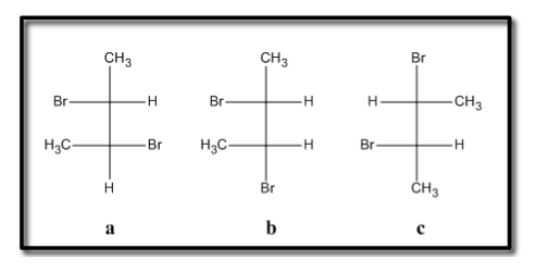# Problem: Which of the following statements is (are) true about compounds a, b and c drawn below?   a. a and b are enantiomers of each other b. b and c are diastereomers of each other c. an equal mixture of a and b is optically active d. an equal mixture of a and c is optically active e. both b and c are true statements

🤓 Based on our data, we think this question is relevant for Professor Daoudi's class at UCF.

###### Problem Details

Which of the following statements is (are) true about compounds a, b and c drawn below?

a. a and b are enantiomers of each other

b. b and c are diastereomers of each other

c. an equal mixture of a and b is optically active

d. an equal mixture of a and c is optically active

e. both b and c are true statementsWhat scientific concept do you need to know in order to solve this problem?

Our tutors have indicated that to solve this problem you will need to apply the Optical Activity concept. You can view video lessons to learn Optical Activity. Or if you need more Optical Activity practice, you can also practice Optical Activity practice problems.

What is the difficulty of this problem?

Our tutors rated the difficulty ofWhich of the following statements is (are) true about compou...as high difficulty.

How long does this problem take to solve?

Our expert Organic tutor, Johnny took 7 minutes and 39 seconds to solve this problem. You can follow their steps in the video explanation above.

What professor is this problem relevant for?

Based on our data, we think this problem is relevant for Professor Daoudi's class at UCF.